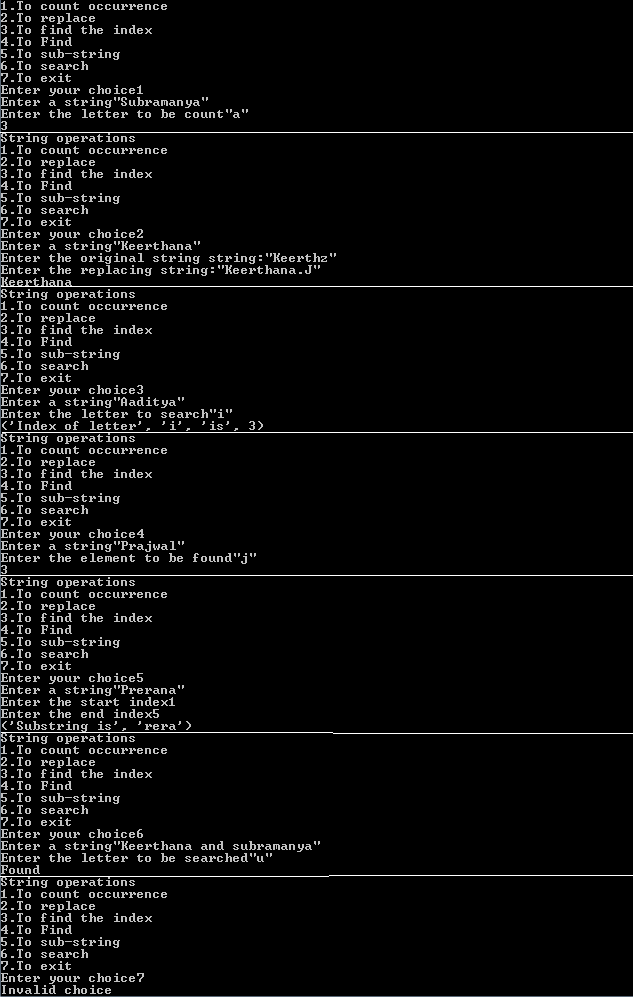# Perform various methods on strings in Python

This post is about a menu based program to perform various methods on strings using Python.

So in this Python program, we are going to include counting occurrence, to replace, to search, to find the index, to find, to sub-string and finally exit from the program. To exit from the menu based at the end of the program, we should make sure that in the beginning of the program we import “import sys“.

This program will be demonstrated using while loop. Talking about the while loop in Python, let us discuss briefly about how while loop works. The syntax of a while loop in Python programming is − while (expression): statement.

Here, statement could be single statement or a block of statements. And the expression can take only two values and those two values are Boolean expression (True or False) also we must make sure that the first letter of the boolean expression(True or False) must be in capital and rest all in small.

Now see our program below before continue:

```import sys
while(True):
print("String operations")
print("1.To count occurrence")
print("2.To replace")
print("3.To find the index")
print("4.To Find")
print("5.To sub-string")
print("6.To search")
print("7.To exit")
if ch==1:
str=input("Enter a string")
a=input("Enter the letter to be count")
print(str.count(a))
elif ch==2:
str=input("Enter a string")
a=input("Enter the original string string:")
b=input("Enter the replacing string:")
print(str.replace(a,b))
elif ch==3:
str=input("Enter a string")
a=input("Enter the letter to search")
print("Index of letter",a,"is",str.index(a))
elif ch==4:
str=input("Enter a string")
a=input("Enter the element to be found")
print(str.find(a))
elif ch==5:
str=input("Enter a string")
a=input("Enter the start index")
b=input("Enter the end index")
print("Substring is",str[a:b])
elif ch==6:
str=input("Enter a string")
a=input("Enter the letter to be searched")
if(a in str):
print("Found")
else:
else:
print("Invalid choice")
sys.exit()```

Below is the output:Explanation:

In the below program we have performed the string methods/operations.

Firstly with to count occurrence, it tells us about how many times the letter we have entered has repeated, Secondly we see to replace, this will replace the original string with new string, thirdly we come up with to find the index, and this tells us at what position is the letter present at.

Also, read: How to unpack a tuple in Python

Furthermore, we see to find, this is similar to the index function and tells us about the position of the letter. Later we see to sub-string, this take the starting index from the given string and end index of the given string and it will trim the string according to the input given, lastly we see to search, this will tell us whether the letter is being present in the string or not.

Note: While taking the output make sure that you enter the strings within double quotes, because we have used only input() and not raw_input(). The difference between input() and raw_input() is, input() takes the value of integer and string value in double-quotes but in raw_input() there won’t be any necessity of giving the values in double-quotes.

### 3 responses to “Perform various methods on strings in Python”

1.Adithya says:

Very informative!

2.Subramanya says:

It’s seems like you are topper and highly educated

3.BLADE CENA says:

Awesome contents